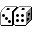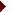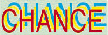##Mathematical Appendix to the Maclean's Magazine article by John Schofield, November 4, 1996

### General Notes

John Schofield's article in the Personal Finance section quotes a number of calculations which I provided to Maclean's. The expectations were based on the payoffs of the September 7, 1996 lottery for which the jackpot prize was \$10,000,000. As that figure was quite unusual I used the amount \$2,217,321 which was the jackpot a week earlier, and which is a more typical figure. This web site will attempt to provide some useful information concerning Lotto, both probability calculations and strategies. The site will be active indefinitely and will grow as additional information and sources are included and as the information is updated. Please bookmark this site and feel free to provide any feedback or to contact me if you have questions.

### Probability BackgroundThe theory of probability is a mathematical model for understanding, describing, analyzing, and predicting phenomena which exhibit random fluctuations in their behaviour. These are characterized by the property that small scale prediction cannot be achieved with high accuracy, but there is remarkable long-run regularity which can be studied mathematically. While the subject has roots in games of chance (in particular in a correspondence between the philosopher Blaise Pascal and a French nobleman the Chevalier de Mere) it has developed far beyond to address fundamental issues in genetics, physics, medicine, communications theory, and software reliability, for instance.

There are three types of probabilities, each differing only in interpretation but still subject to the same rules:

Mathematical
The theoretical development based on equally likely outcomes and their far-reaching generalization into measure theory.
Empirical
Events are assigned probabilities based on empirical observations from the past.
Subjective
This was championed by the Italian mathematician Bruno de Finetti and views probabilities as personal reflections of an individual's opinion about an event.
The probabilities associated with Lotto 6/49 are determined by calculations based on equally likely outcomes but their interpretation is empirical, as follows. If an experiment (such as a weekly Lotto drawing) is repeated under similar conditions many times (mathematically the requisite number of times must approach infinity) then the probability of an event is the long run proportion of experimental repetitions on which the event occurs. (This is called a law of large numbers. It is a mathematical result only.) As illustration, the probability of obtaining a fourth place prize is 0.0009686. This means that in 26,000 Lotto draws, each particular choice of six numbers will yield a fourth place prize (matching exactly 4 numbers) on approximately 0.0009686 of those plays, that is 26000 x 0.000986 = 25 times. There is no guarantee that this will happen 25 times. The actual number of times is random and subject to what is called the Poisson approximation. The figure of 25 represents an expected number of winnings and is a useful measure by which to compare the performance of different strategies.

### How the Probabilities are CalculatedThe following discussion is a very brief description of the combinatorics needed to derive the Lotto 6/49 probabilities.Jackpot (all six winning numbers selected)
There are a total of 13,983,816 different groups of six numbers which could be drawn from the set {1, 2, ... , 49}. To see this we observe that there are 49 possibilities for the first number drawn, following which there are 48 possibilities for the second number, 47 for the third, 46 for the fourth, 45 for the fifth, and 44 for the sixth. If we multiply the numbers 49 x 48 x 47 x 46 x 45 x 44 we get 10,068,347,520. However, each possible group of six numbers (combination) can be drawn in different ways depending on which number in the group was drawn first, which was drawn second, and so on. There are 6 choices for the first, 5 for the second, 4 for the third, 3 for the fourth, 2 for the fifth, and 1 for the sixth. Multiply these numbers out to arrive at 6 x 5 x 4 x 3 x 2 x 1 = 720. We then need to divide 10,068,347,520 by 720 to arrive at the figure 13,983,816 as the number of different groups of six numbers (different picks). Since all numbers are assumed to be equally likely and since the probability of some number being drawn must be one, it follows that each pick of six numbers has a probability of 1/13,983,816 = 0.00000007151. This is roughly the same probability as obtaining 24 heads in succession when flipping a fair coin!Second Prize (five winning numbers + bonus)
The pick of six must include 5 winning numbers plus the bonus. Since 5 of the six winning numbers must be picked, this means that one of the winning numbers must be excluded. There are six possibilities for the choice of excluded number and hence there are six ways for a pick of six to win the second place prize. The probability is thus 6/13,983,816 = 0.0000004291 which translates into odds against of 2,330,635:1.Third Prize (five winning numbers selected, bonus number not selected)
As in the second prize there are six ways for a pick of six to include exactly five of the six drawn numbers. The remaining number must be one of the 42 numbers left over after the six winning numbers and the bonus number have been excluded. Thus there are a total of 6 x 42 = 252 ways for a pick of six to win the third prize. This becomes a probability of 252/13,983,816 = 0.00001802 or, equivalently, odds against of 55490.3:1.Fourth Prize (four winning numbers selected)
There are 15 ways to include four of the six winning numbers and 903 ways to include two of the 43 non-winning numbers for a total of 15 x 903 = 13,545 ways for a pick of six to win the fourth prize, which works out to a probability of 13,545/13,983,816 = 0.0009686, that is odds against of 1031.4:1.Fifth Prize (three winning numbers selected)
There are 20 ways to include three of the six winning numbers and 12,341 ways to include three of the 43 non-winning numbers for a total of 20 x 12,341 = 246,820 ways for a pick of six to win the fifth prize, which works out to a probability of 246,820/13,983,816 = 0.01765, that is odds against of 55.7:1.

### Other Sources of InformationA wonderful source of statistical information as it applies to everyday life, including lotteries, is the Chance Project at Dartmouth University.